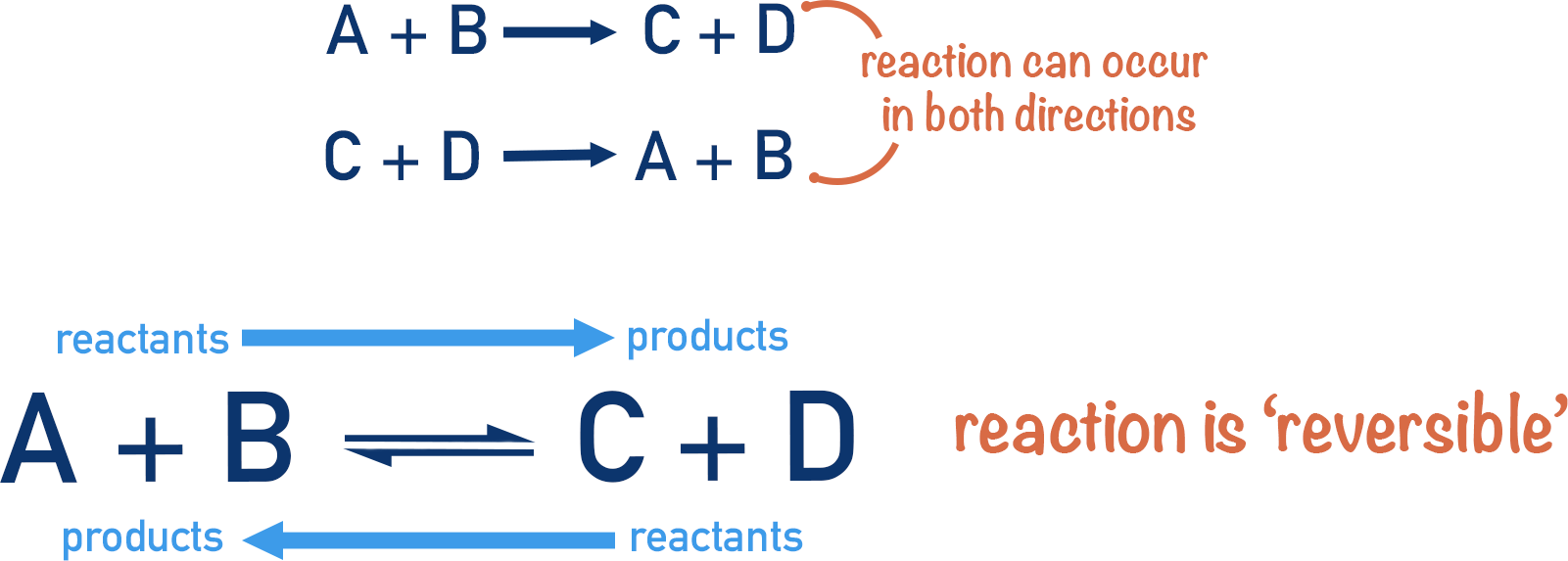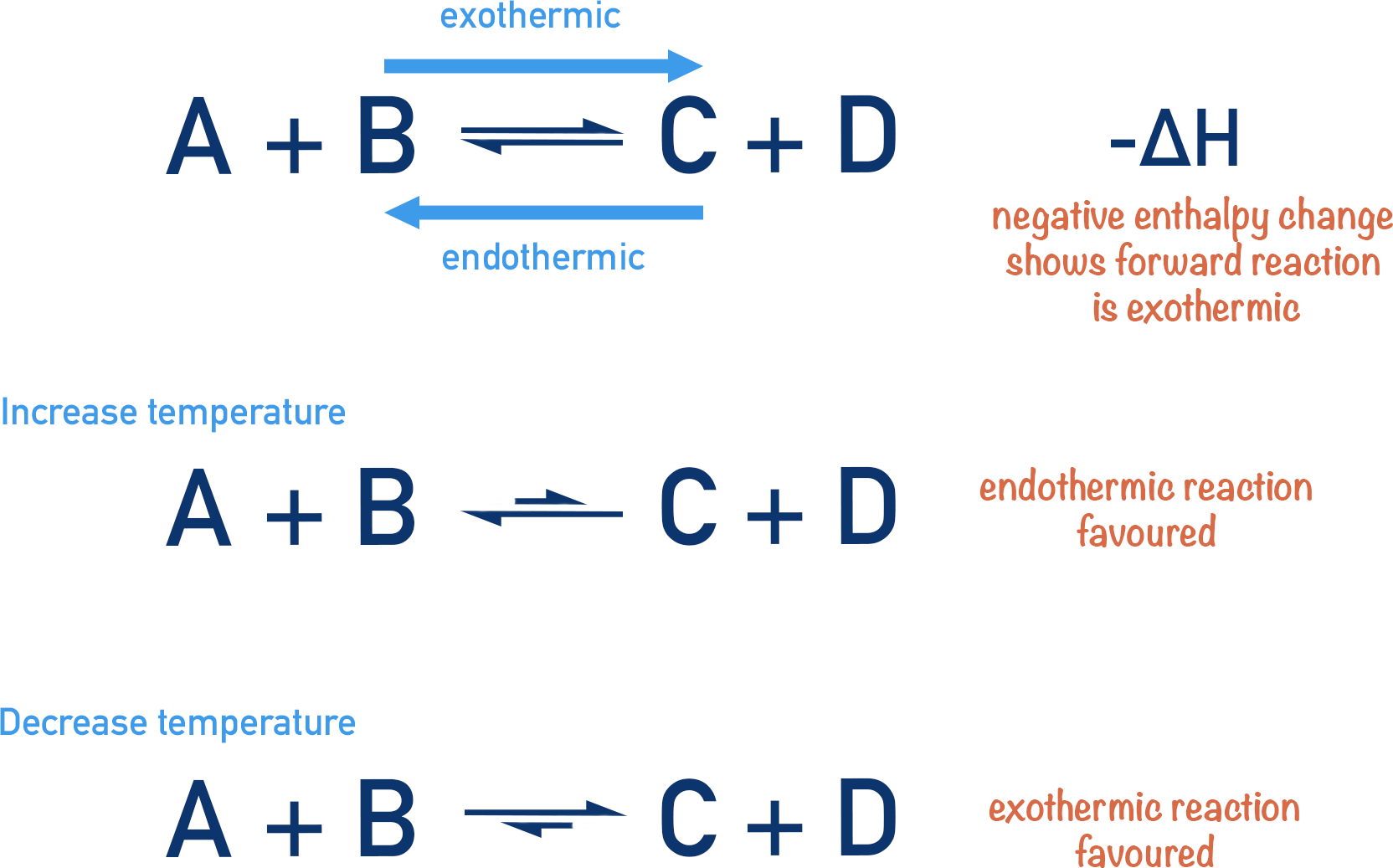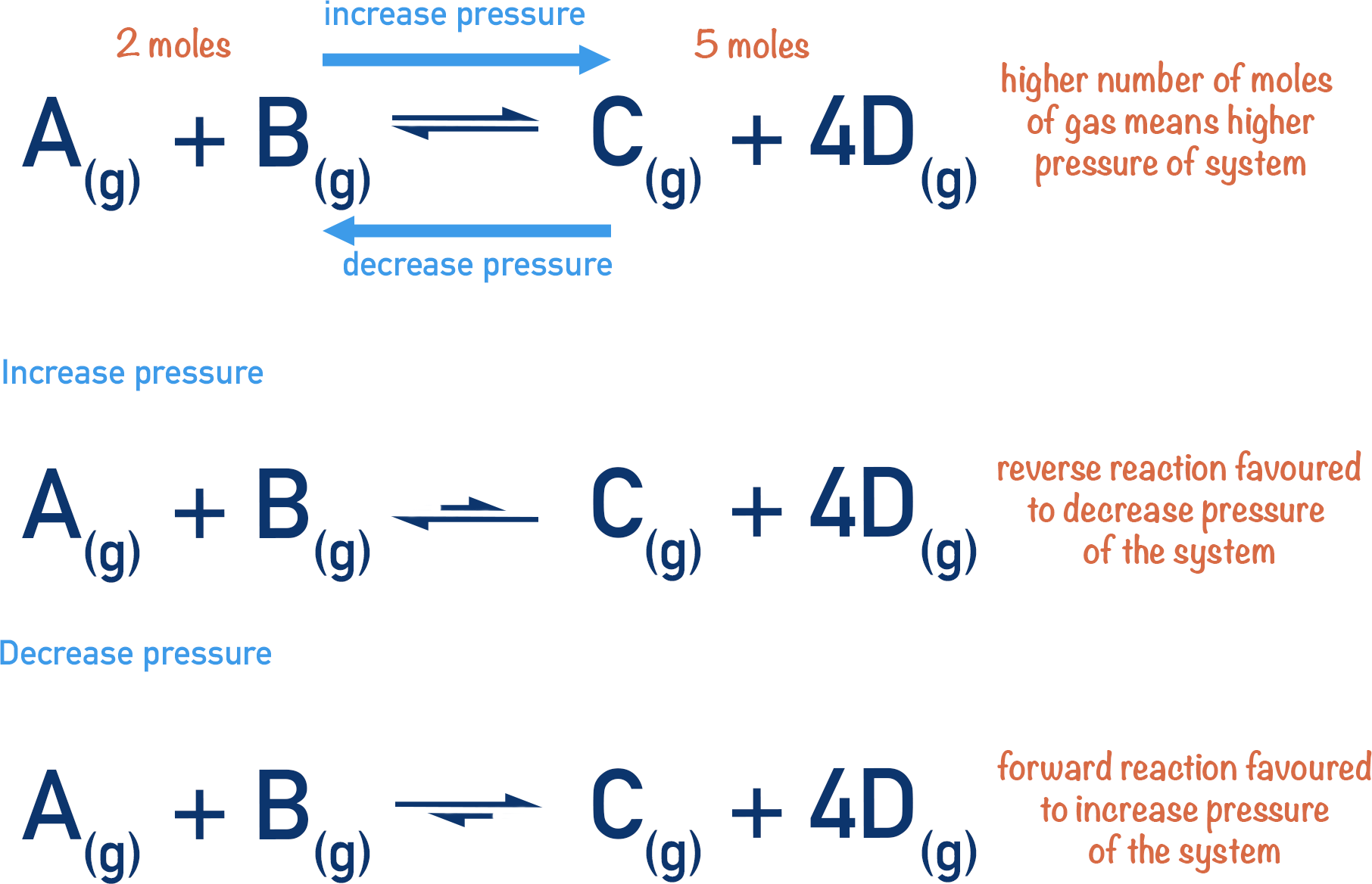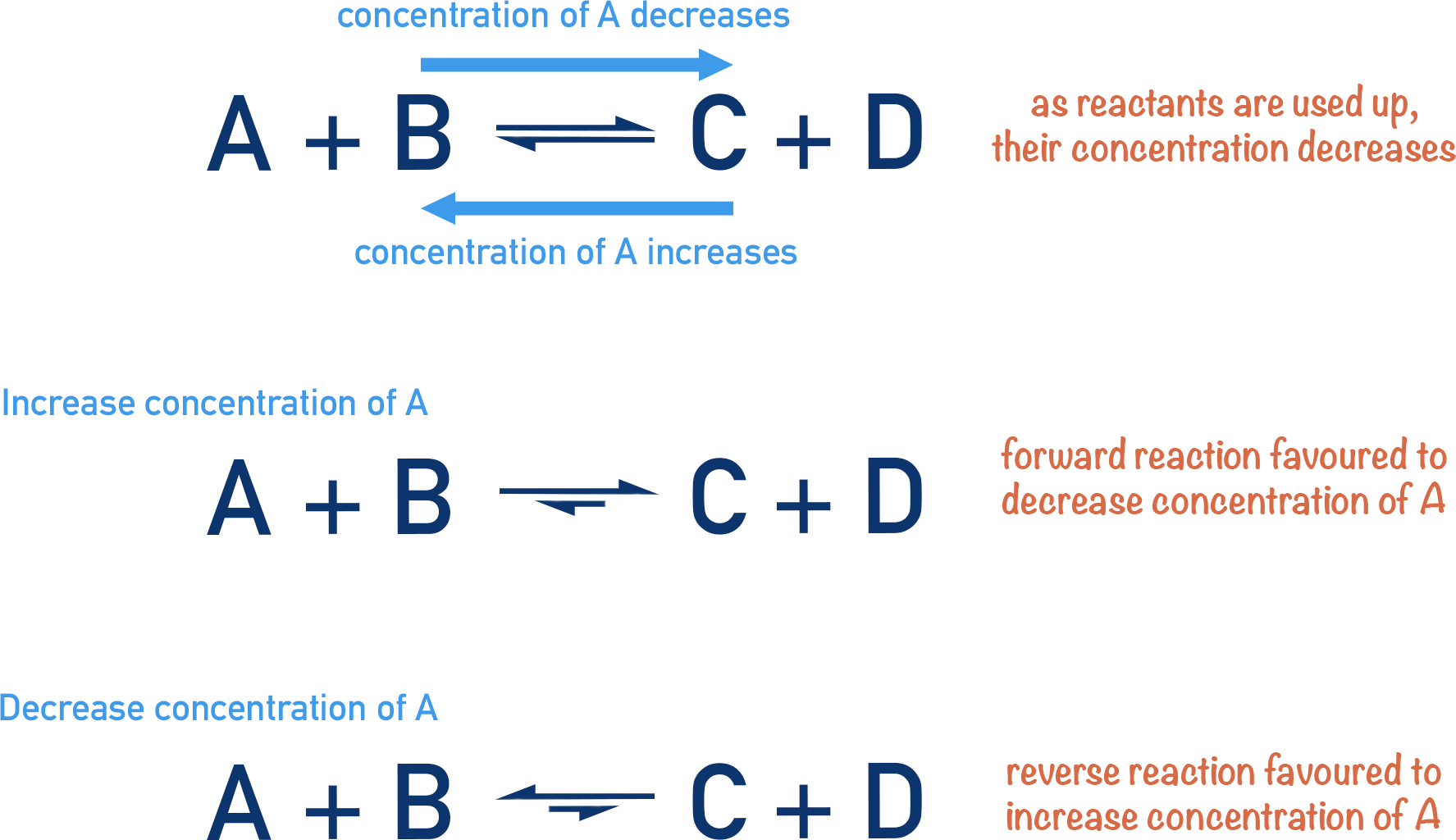Video Tutorial Equilibrium

Quick Notes Equilibrium

• In a reversible reaction, reactants react to produce products (forward reaction) and those products also react to produce the original reactants (reverse reaction).
• At equilibrium, the rates of both the forward and reverse reactions are the same – the concentrations of all reactants and products do not change.
• The position of equilibrium describes how much one reaction has been favoured compared to the other before equilibrium is reached.
• Changing the conditions of an equilibrium system can cause the position of equilibrium to move.
• Le Chatelier’s Principle states that the position of equilibrium will move to oppose a change in conditions.
• Increasing the temperature of a system forces the position of equilibrium to move to favour the endothermic reaction.
• Increasing the concentration of a reactant forces the position of equilibrium to move to favour the reaction that uses up that reactant.
• Increasing the pressure of a system forces the position of equilibrium to move to favour the reaction that produces the fewest moles of gas.

Full Notes Equilibrium

Some reactions are reversible. In a reversible reaction, reactants are reacting to produce new products, whilst at the same time, these products are also reacting to produce the original reactants.

Imagine it as being a bit like a motorway – cars are driving to London from Birmingham. At the same time, however, cars are also driving from London to Birmingham.When these two reactions are happening at the same rate, we say the reactions are at equilibrium. The number of cars heading into London from Birmingham is the same as the number of cars leaving London going into Birmingham.

At equilibrium, because the reactants are being used-up at the same rate as they are being produced, the concentrations of the reactants and the products do not change. Remember these reactions are still happening!

The position of equilibrium describes how much one reaction has been favoured compared to the other before equilibrium is reached. A bit like how many more cars went to London than Birmingham, before the numbers going in and out of both became the same.

## Le Chatelier’s Principle

A French chemist, Le Chatelier, discovered that we can change how much of each reaction is occurring.

He stated that the position of equilibrium will always move to oppose any change to an equilibrium system. This just means that the equilibrium will always want to do the opposite of whatever is being done to the system.

If, for example, the temperature is increased, the position of equilibrium will move to decrease the temperature.

If the pressure in a gaseous system is increased, the position of equilibrium will move to decrease the pressure, and so on.

This principle can be very useful for predicting what will happen in certain conditions and how the positions of equilibrium can be tweaked to produce as much desired product as possible.

## Effect of Temperature Change on Equilibrium

Reactions have an energy change: they are either exothermic (release energy in the form of heat) or endothermic (take in energy in the form of heat). With a reversible reaction, there are two reactions that are basically opposites of themselves. So, if in one direction the reaction is exothermic, in the other it must be endothermic.

Exothermic reactions release energy to the surroundings, increasing the temperature of the equilibrium system.

Endothermic reactions take in energy from the surroundings, decreasing the temperature of the equilibrium system.If the temperature of an equilibrium system is increased, Le Chatelier’s principle tells us that the position of equilibrium will move to oppose that increase in temperature. This means the position of equilibrium will move to favour the endothermic reaction – effectively ‘removing’ the increased temperature (lowering the temperature of the equilibrium system).

If the temperature of an equilibrium system is decreased, the opposite is true. The position of equilibrium will move to favour the exothermic reaction.

## Effect of Pressure Change on Equilibrium

In a homogenous (all substances are in the same state) gaseous equilibrium system, if the pressure is changed, the position of equilibrium will move to oppose this change.

In A-level Chemistry, we assume that all gases occupy the same volume at a given temperature and pressure – irrespective of their individual size. This means that 1 mole of methane (CH4) would occupy the same amount of space as 1 mole of butane (C4H10). Even though butane is actually four times the size of methane, the difference in physical size of a molecule is very small compared to the volume gases occupy. See Moles – Ideal Gases.

If the pressure of an equilibrium system is increased, the position of equilibrium will move to favour the reaction that produces the least number of gaseous molecules and lowers the pressure of the system. If the pressure is increased, the reaction that has the least number of gaseous products will be favoured.If the pressure of the system is decreased, the opposite is true. The reaction that produces the greatest number of moles of gas will be favoured.

## Effect of Concentration Change on Equilibrium

If the concentration of a reactant (or product) is changed, then how much of that reactant is present also changes. Le Chatelier’s principle tells us that the position of equilibrium will move to oppose a change. So, if the concentration of a reactant is increased, the position of equilibrium will move to lower its concentration. This is achieved by favouring the reaction that uses up that reactant. If the concentration of a reactant is decreased, the reaction that produces this reactant will be favoured, increasing its concentration and opposing the change.## Effect of a Catalyst on Equilibrium

A catalyst does not have any effect on the position of equilibrium. A catalyst just changes the rates of each reaction and both reactions are affected in equal amounts.